## Machine Learning

Certification Training
15117 Learners
7 Chapters +

# Unsupervised Learning with Clustering - Machine Learning

This is ‘Unsupervised Learning with Clustering’ tutorial which is a part of the Machine Learning course offered by Simplilearn. We will learn machine learning clustering algorithms and K-means clustering algorithm majorly in this tutorial.

## Objectives

Let us look at the objectives covered in this Clustering Tutorial.

• Discuss machine learning clustering algorithms
• Explain the k-means clustering algorithm with examples

## Recall: Clustering

Cluster analysis or clustering is the most commonly used technique of unsupervised learning. It is used to find data clusters such that each cluster has the most closely matched data.

## Clustering Algorithms

The types of Clustering Algorithms are:

• Prototype-based Clustering
• Hierarchical Clustering
• Density-based Clustering (DBSCAN)

### Prototype-based Clustering

Prototype-based clustering assumes that most data is located near prototypes; example: centroids (average) or medoid (most frequently occurring point) K-means, a Prototype-based method, is the most popular method for clustering that involves:

• Training data that gets assigned to matching cluster based on similarity
• The iterative process to get data points in the best clusters possible

## What is K-means Clustering ?

K-means clustering is an unsupervised learning algorithm. In this case, you don't have labeled data unlike in supervised learning. You have a set of data that you want to group into and you want to put them into clusters, which means objects that are similar in nature and similar in characteristics need to be put together. This is what k-means clustering is all about. The term K is basically is a number and you need to tell the system how many clusters you need to perform. If K is equal to 2, there will be 2 clusters if K is equal to 3, 3 clusters and so on and so forth. That's what the K stands for and of course, there is a way of finding out what is the best or optimum value of K.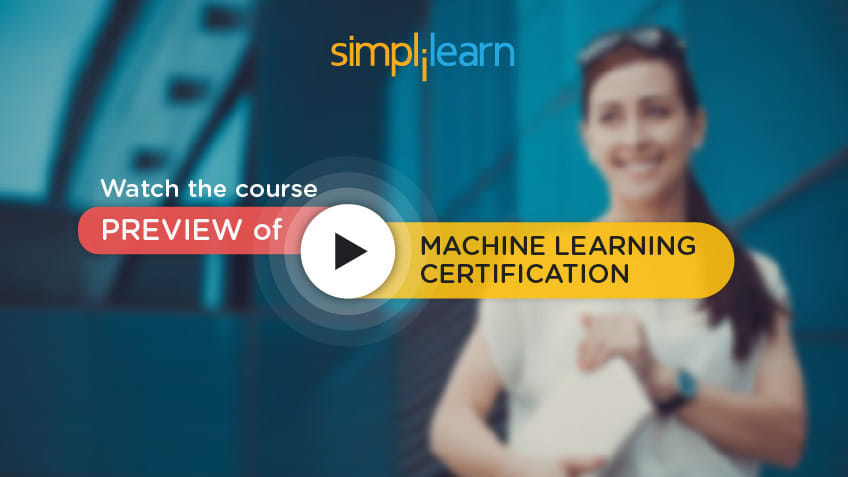## K-means Clustering example

Let us understand K-means clustering examples below.

### Problem Statement

Let’s say, in California, the government tries to identify high-density clusters to build hospitals (no other ground truth or features are provided apart from the population data). How can the clusters be identified?

Step 1: Randomly Pick K Centroids

Start by picking k centroids. Assume, k = 3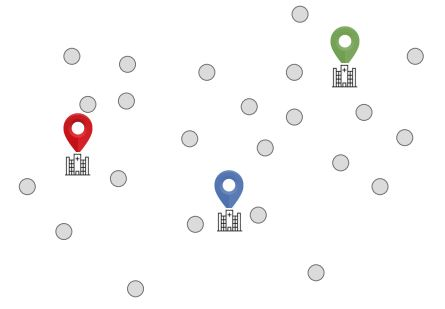Finding the number of clusters: Use Elbow Method (to be reviewed later)

Step 2: Assign Each Point To The Nearest Centroid Μ(J), J ∈ {1,…, K}.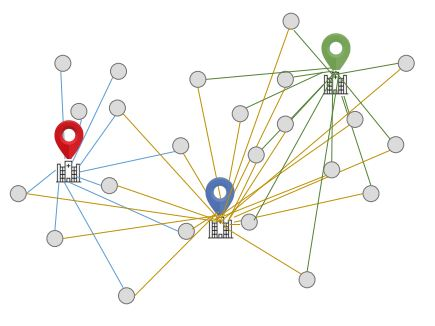The points are assigned such that the Euclidean distance of each point from the respective centroid is minimized

Step 3: Move Each Centroid To The Centre Of The Respective ClusterStep 4: Calculate Distance Of The Centroids From Each Point AgainCalculate the Euclidean distance between each point and its centroid.

Step 5: Move Points Across Clusters And Re-calculate The Distance From The Centroid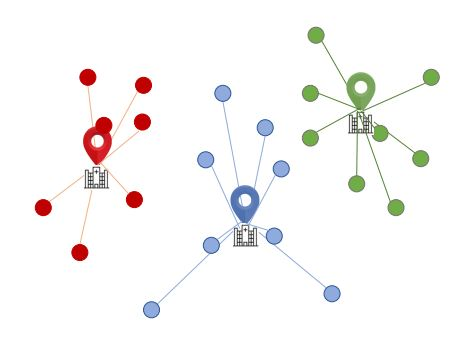Step 6: Keep Moving The Points Across Clusters Until The Euclidean Distance Is MinimizedRepeat the steps until the within-cluster Euclidean distance is minimized for each cluster (or a user-defined limit on the number of iterations is reached)

### Giving it a Mathematical Angle

The analysis was based on a lot of calculations. Now let’s understand the mathematical aspect.

• A key challenge in Clustering is that you have to pre-set the number of clusters. This influences the quality of clustering.
• Unlike Supervised Learning, here one does not have ground truth labels. Hence, to check the quality of clustering, one has to use intrinsic methods, such as the within-cluster SSE, also called Distortion.
• In the scikit-learn ML library, this value is available via the inertia_ attribute after fitting a K-means model.
• One could plot the Distortion against the number of clusters k. Intuitively, if k increases, distortion should decrease. This is because the samples will be close to their assigned centroids.
• This plot is called the Elbow method. It indicates the optimum number of clusters at the position of the elbow, the point where distortion begins to increase most rapidly
• The adjoining Elbow method suggests that k = 3 is the most optimum number of clusters.• K-means is based on finding points close to cluster centroids. The distance between two points x and y can be measured by the squared Euclidean distance between them in an m-dimensional space.
• Here, j refers to j-th dimension (or j-th feature) of the data point.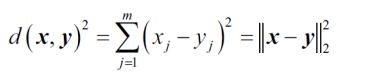• Based on this, the optimization problem is to minimize the within-cluster sum of squared errors (SSE), which is sometimes also called the cluster inertia.
• Here, j refers to the j-th cluster. μ (j) is the centroid of that cluster.
• w(i,j) = 1 if the sample x (i) is in cluster j, and 0 otherwise.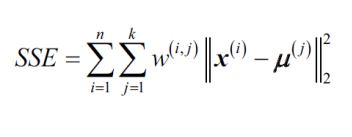## Mathematical Representation(Contd.)

Scikit-learn cluster module has the K-means function. In the code shown,

• k = 3
• n_init = 10: which means that you run the clustering logic 10 times, each time with random cluster centroids. Finally, the model with the lowest SSE among the 10 schemes gets chosen.
• max_iter = 300: which means within each of the 10 runs, iterate 300 times to find ideal clusters.
• If convergence happens before 300 iterations, it will stop early.
• A large max_iter is computationally intensive (if convergence does not happen early).
• tol is another parameter which governs tolerance with regard to changes in the within-cluster SSE to declare convergence. A larger tol means it will declare convergence sooner.

## Other K-means Clustering Examples

Some of the examples related to K-means Clustering.

• Grouping articles (example: Google news)
• Grouping customers who share similar interests, for example: analyzing customers who like contemporary fashion vs. those who prefer traditional clothing
• Classifying high risk and low-risk patients from a patient pool
• Segregating criminals from the normal crowd in a security control process

## Key Takeaways

Let us quickly go through what you have learned so far in this tutorial.

• The most common form of Unsupervised Learning is Clustering, which involves segregating data based on the similarity between data instances.
• K-means is a popular technique for Clustering. It involves an iterative process to find cluster centers called centroids and assigning data points to one of the centroids.
• K-means finds clusters by minimizing the within-cluster distance of data points from respective centroids.
• The Elbow method is used to determine the most optimum number of clusters.

This concludes “Unsupervised Learning with Clustering.” With this we come to an end to the Machine Learning Tutorial.

### Find our Machine Learning Online Classroom training classes in top cities:

Name Date Place
Machine Learning 27 Feb -3 Apr 2021, Weekend batch Your City View Details
Machine Learning 8 Mar -26 Mar 2021, Weekdays batch New York City View Details
Machine Learning 12 Mar -16 Apr 2021, Weekdays batch San Francisco View Details
Related Courses
Learner Reviews
Related Articles
• Disclaimer
• PMP, PMI, PMBOK, CAPM, PgMP, PfMP, ACP, PBA, RMP, SP, and OPM3 are registered marks of the Project Management Institute, Inc.

Name*
Email*
Phone Number*